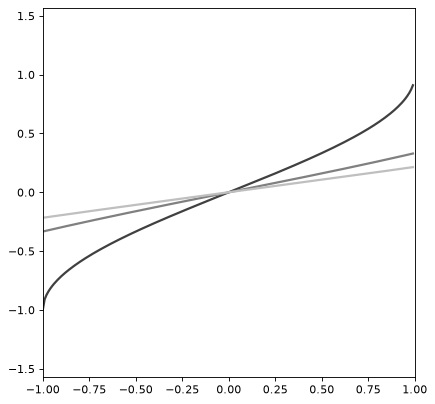# ArcSine1D¶

class astropy.modeling.functional_models.ArcSine1D(*args, meta=None, name=None, **kwargs)[source]

Bases: astropy.modeling.functional_models._InverseTrigonometric1D

One dimensional ArcSine model returning values between -pi/2 and pi/2 only.

Parameters
amplitudefloat

Oscillation amplitude for corresponding Sine

frequencyfloat

Oscillation frequency for corresponding Sine

phasefloat

Oscillation phase for corresponding Sine

Other Parameters
fixeda dict, optional

A dictionary {parameter_name: boolean} of parameters to not be varied during fitting. True means the parameter is held fixed. Alternatively the fixed property of a parameter may be used.

tieddict, optional

A dictionary {parameter_name: callable} of parameters which are linked to some other parameter. The dictionary values are callables providing the linking relationship. Alternatively the tied property of a parameter may be used.

boundsdict, optional

A dictionary {parameter_name: value} of lower and upper bounds of parameters. Keys are parameter names. Values are a list or a tuple of length 2 giving the desired range for the parameter. Alternatively, the min and max properties of a parameter may be used.

eqconslist, optional

A list of functions of length n such that eqcons[j](x0,*args) == 0.0 in a successfully optimized problem.

ineqconslist, optional

A list of functions of length n such that ieqcons[j](x0,*args) >= 0.0 is a successfully optimized problem.

Notes

Model formula:

$f(x) = ((arcsin(x / A) / 2pi) - p) / f$

The arcsin function being used for this model will only accept inputs in [-A, A]; otherwise, a runtime warning will be thrown and the result will be NaN. To avoid this, the bounding_box has been properly set to accommodate this; therefore, it is recommended that this model always be evaluated with the with_bounding_box=True option.

Examples

import numpy as np
import matplotlib.pyplot as plt

from astropy.modeling.models import ArcSine1D

plt.figure()
s1 = ArcSine1D(amplitude=1, frequency=.25)
r=np.arange(-1, 1, .01)

for amplitude in range(1,4):
s1.amplitude = amplitude
plt.plot(r, s1(r), color=str(0.25 * amplitude), lw=2)

plt.axis([-1, 1, -np.pi/2, np.pi/2])
plt.show()


(png, svg, pdf)Methods Summary

 evaluate(x, amplitude, frequency, phase) One dimensional ArcSine model function fit_deriv(x, amplitude, frequency, phase) One dimensional ArcSine model derivative

Methods Documentation

static evaluate(x, amplitude, frequency, phase)[source]

One dimensional ArcSine model function

static fit_deriv(x, amplitude, frequency, phase)[source]

One dimensional ArcSine model derivative